# Platonic solid

﻿
Platonic solid

In geometry, a Platonic solid is a convex polyhedron that is regular, in the sense of a regular polygon. Specifically, the faces of a Platonic solid are congruent regular polygons, with the same number of faces meeting at each vertex; thus, all its edges are congruent, as are its vertices and angles.

There are five Platonic solids (shown below):

The name of each figure is derived from its number of faces: respectively 4, 6, 8, 12, and 20.

The aesthetic beauty and symmetry of the Platonic solids have made them a favorite subject of geometers for thousands of years. They are named for the ancient Greek philosopher Plato who theorized that the classical elements were constructed from the regular solids. With duality, truncation, and snubbing, the Tetrahedron first forms the other triangular platonic solids, then duality makes the other two.

## History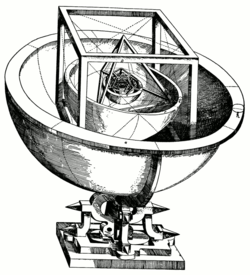Kepler's Platonic solid model of the solar system from Mysterium Cosmographicum (1596)

The Platonic solids have been known since antiquity. Ornamented models of them can be found among the carved stone balls created by the late neolithic people of Scotland at least 1000 years before Plato (Atiyah and Sutcliffe 2003). Dice go back to the dawn of civilization with shapes that augured formal charting of Platonic solids.

The ancient Greeks studied the Platonic solids extensively. Some sources (such as Proclus) credit Pythagoras with their discovery. Other evidence suggests he may have only been familiar with the tetrahedron, cube, and dodecahedron, and that the discovery of the octahedron and icosahedron belong to Theaetetus, a contemporary of Plato. In any case, Theaetetus gave a mathematical description of all five and may have been responsible for the first known proof that there are no other convex regular polyhedra.

The Platonic solids feature prominently in the philosophy of Plato for whom they are named. Plato wrote about them in the dialogue Timaeus c.360 B.C. in which he associated each of the four classical elements (earth, air, water, and fire) with a regular solid. Earth was associated with the cube, air with the octahedron, water with the icosahedron, and fire with the tetrahedron. There was intuitive justification for these associations: the heat of fire feels sharp and stabbing (like little tetrahedra). Air is made of the octahedron; its minuscule components are so smooth that one can barely feel it. Water, the icosahedron, flows out of one's hand when picked up, as if it is made of tiny little balls. By contrast, a highly un-spherical solid, the hexahedron (cube) represents earth. These clumsy little solids cause dirt to crumble and break when picked up, in stark difference to the smooth flow of water. Moreover, the solidity of the Earth was believed to be due to the fact that the cube is the only regular solid that tesselates Euclidean space. The fifth Platonic solid, the dodecahedron, Plato obscurely remarks, "...the god used for arranging the constellations on the whole heaven". Aristotle added a fifth element, aithêr (aether in Latin, "ether" in English) and postulated that the heavens were made of this element, but he had no interest in matching it with Plato's fifth solid.[citation needed]

Euclid gave a complete mathematical description of the Platonic solids in the Elements, the last book (Book XIII) of which is devoted to their properties. Propositions 13–17 in Book XIII describe the construction of the tetrahedron, octahedron, cube, icosahedron, and dodecahedron in that order. For each solid Euclid finds the ratio of the diameter of the circumscribed sphere to the edge length. In Proposition 18 he argues that there are no further convex regular polyhedra. Andreas Speiser has advocated the view that the construction of the 5 regular solids is the chief goal of the deductive system canonized in the Elements. Much of the information in Book XIII is probably derived from the work of Theaetetus.

In the 16th century, the German astronomer Johannes Kepler attempted to find a relation between the five extraterrestrial planets known at that time and the five Platonic solids. In Mysterium Cosmographicum, published in 1596, Kepler laid out a model of the solar system in which the five solids were set inside one another and separated by a series of inscribed and circumscribed spheres. The six spheres each corresponded to one of the planets (Mercury, Venus, Earth, Mars, Jupiter, and Saturn). The solids were ordered with the innermost being the octahedron, followed by the icosahedron, dodecahedron, tetrahedron, and finally the cube. In this way the structure of the solar system and the distance relationships between the planets was dictated by the Platonic solids. In the end, Kepler's original idea had to be abandoned, but out of his research came the recognition that the orbits of planets are ellipses rather than circles, as well as his two laws of orbital dynamics, changing the courses of physics and astronomy, plus the discovery of the Kepler solids.

## Combinatorial properties

A convex polyhedron is a Platonic solid if and only if

1. all its faces are congruent convex regular polygons,
2. none of its faces intersect except at their edges, and
3. the same number of faces meet at each of its vertices.

Each Platonic solid can therefore be denoted by a symbol {p, q} where

p = the number of edges of each face (or the number of vertices of each face) and
q = the number of faces meeting at each vertex (or the number of edges meeting at each vertex).

The symbol {p, q}, called the Schläfli symbol, gives a combinatorial description of the polyhedron. The Schläfli symbols of the five Platonic solids are given in the table below.

Polyhedron Vertices Edges Faces Schläfli symbol Vertex
configuration
Historically corresponding element
tetrahedron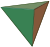4 6 4 {3, 3} 3.3.3 Fire
cube / hexahedron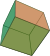8 12 6 {4, 3} 4.4.4 Earth
octahedron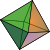6 12 8 {3, 4} 3.3.3.3 Air
dodecahedron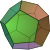20 30 12 {5, 3} 5.5.5 Quintessence / Universe
icosahedron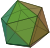12 30 20 {3, 5} 3.3.3.3.3 Water

All other combinatorial information about these solids, such as total number of vertices (V), edges (E), and faces (F), can be determined from p and q. Since any edge joins two vertices and has two adjacent faces we must have:$pF = 2E = qV.\,$

The other relationship between these values is given by Euler's formula:$V - E + F = 2.\,$

This nontrivial fact can be proved in a great variety of ways (in algebraic topology it follows from the fact that the Euler characteristic of the sphere is 2). Together these three relationships completely determine V, E, and F:$V = \frac{4p}{4 - (p-2)(q-2)},\quad E = \frac{2pq}{4 - (p-2)(q-2)},\quad F = \frac{4q}{4 - (p-2)(q-2)}.$

Note that swapping p and q interchanges F and V while leaving E unchanged (for a geometric interpretation of this fact, see the section on dual polyhedra below).

## Classification

It is a classical result that there are only five convex regular polyhedra. Two common arguments are given below. Both of these arguments only show that there can be no more than five Platonic solids. That all five actually exist is a separate question—one that can be answered by an explicit construction.

### Geometric proof

The following geometric argument is very similar to the one given by Euclid in the Elements:

1. Each vertex of the solid must coincide with one vertex each of at least three faces.
2. At each vertex of the solid, the total, among the adjacent faces, of the angles between their respective adjacent sides must be less than 360°.
3. The angles at all vertices of all faces of a Platonic solid are identical, so each vertex of each face must contribute less than 360°/3 = 120°.
4. Regular polygons of six or more sides have only angles of 120° or more, so the common face must be the triangle, square, or pentagon. And for:
• Triangular faces: each vertex of a regular triangle is 60°, so a shape may have 3, 4, or 5 triangles meeting at a vertex; these are the tetrahedron, octahedron, and icosahedron respectively.
• Square faces: each vertex of a square is 90°, so there is only one arrangement possible with three faces at a vertex, the cube.
• Pentagonal faces: each vertex is 108°; again, only one arrangement, of three faces at a vertex is possible, the dodecahedron.

### Topological proof

A purely topological proof can be made using only combinatorial information about the solids. The key is Euler's observation that VE + F = 2, and the fact that pF = 2E = qV, where p stands for the number of edges of each face and q for the number of edges meeting at each vertex. Combining these equations one obtains the equation$\frac{2E}{q} - E + \frac{2E}{p} = 2.$

Simple algebraic manipulation then gives${1 \over q} + {1 \over p}= {1 \over 2} + {1 \over E}.$

Since E is strictly positive we must have$\frac{1}{q} + \frac{1}{p} > \frac{1}{2}.$

Using the fact that p and q must both be at least 3, one can easily see that there are only five possibilities for (p, q):$(3, 3),\quad (4, 3),\quad (3, 4),\quad (5, 3),\quad (3,5).$

## Geometric properties

### Angles

There are a number of angles associated with each Platonic solid. The dihedral angle is the interior angle between any two face planes. The dihedral angle, θ, of the solid {p,q} is given by the formula$\sin{\theta\over 2} = \frac{\cos(\pi/q)}{\sin(\pi/p)}.$

This is sometimes more conveniently expressed in terms of the tangent by$\tan{\theta\over 2} = \frac{\cos(\pi/q)}{\sin(\pi/h)}.$

The quantity h is 4, 6, 6, 10, and 10 for the tetrahedron, cube, octahedron, dodecahedron, and icosahedron respectively.

The angular deficiency at the vertex of a polyhedron is the difference between the sum of the face-angles at that vertex and 2π. The defect, δ, at any vertex of the Platonic solids {p,q} is$\delta = 2\pi - q\pi\left(1-{2\over p}\right).$

By a theorem of Descartes, this is equal to 4π divided by the number of vertices (i.e. the total defect at all vertices is 4π).

The 3-dimensional analog of a plane angle is a solid angle. The solid angle, Ω, at the vertex of a Platonic solid is given in terms of the dihedral angle by$\Omega = q\theta - (q-2)\pi.\,$

This follows from the spherical excess formula for a spherical polygon and the fact that the vertex figure of the polyhedron {p,q} is a regular q-gon.

The solid angle of a face subtended from the center of a platonic solid is equal to the solid angle of a full sphere (4π steradians) divided by the number of faces. Note that this is equal to the angular deficiency of its dual.

The various angles associated with the Platonic solids are tabulated below. The numerical values of the solid angles are given in steradians. The constant φ = (1+√5)/2 is the golden ratio.

Polyhedron Dihedral angle
θ$\tan\frac{\theta}{2}$ Vertex angle Defect (δ) Vertex solid angle (Ω) Face
solid angle
tetrahedron 70.53°$1\over{\sqrt 2}$ 60° π$\cos^{-1}\left(\frac{23}{27}\right)$$\approx 0.551286$ π
cube 90° 1 90°$\pi\over 2$$\frac{\pi}{2}$$\approx 1.57080$$2\pi\over 3$
octahedron 109.47°$\sqrt 2$ 60°, 90°${2\pi}\over 3$$4\sin^{-1}\left({1\over 3}\right)$$\approx 1.35935$$\pi\over 2$
dodecahedron 116.57° φ 108°$\pi\over 5$$\pi - \tan^{-1}\left(\frac{2}{11}\right)$$\approx 2.96174$$\pi\over 3$
icosahedron 138.19° φ2 60°, 108°$\pi\over 3$$2\pi - 5\sin^{-1}\left({2\over 3}\right)$$\approx 2.63455$$\pi\over 5$

Another virtue of regularity is that the Platonic solids all possess three concentric spheres:

The radii of these spheres are called the circumradius, the midradius, and the inradius. These are the distances from the center of the polyhedron to the vertices, edge midpoints, and face centers respectively. The circumradius R and the inradius r of the solid {p, q} with edge length a are given by$R = \left({a\over 2}\right)\tan\frac{\pi}{q}\tan\frac{\theta}{2}$$r = \left({a\over 2}\right)\cot\frac{\pi}{p}\tan\frac{\theta}{2}$

where θ is the dihedral angle. The midradius ρ is given by$\rho = \left({a\over 2}\right)\frac{\cos(\pi/p)}{\sin(\pi/h)}$

where h is the quantity used above in the definition of the dihedral angle (h = 4, 6, 6, 10, or 10). Note that the ratio of the circumradius to the inradius is symmetric in p and q:${R\over r} = \tan\frac{\pi}{p}\tan\frac{\pi}{q}.$

The surface area, A, of a Platonic solid {p, q} is easily computed as area of a regular p-gon times the number of faces F. This is:$A = \left({a\over 2}\right)^2 Fp\cot\frac{\pi}{p}.$

The volume is computed as F times the volume of the pyramid whose base is a regular p-gon and whose height is the inradius r. That is,$V = {1\over 3}rA.$

The following table lists the various radii of the Platonic solids together with their surface area and volume. The overall size is fixed by taking the edge length, a, to be equal to 2.

Polyhedron
(a = 2)
tetrahedron$1\over {\sqrt 6}$$1\over {\sqrt 2}$$\sqrt{3\over 2}$$4\sqrt 3$$\frac{\sqrt 8}{3}$
cube$1\,$$\sqrt 2$$\sqrt 3$$24\,$$8\,$
octahedron$\sqrt{2\over 3}$$1\,$$\sqrt 2$$8\sqrt 3$$\frac{\sqrt {128}}{3}$
dodecahedron$\frac{\varphi^2}{\xi}$ φ2$\sqrt 3\,\varphi$$60\frac{\varphi}{\xi}$$20\frac{\varphi^3}{\xi^2}$
icosahedron$\frac{\varphi^2}{\sqrt 3}$ φ ξφ$20\sqrt 3$$\frac{20\varphi^2}{3}$

The constants φ and ξ in the above are given by$\varphi = 2\cos{\pi\over 5} = \frac{1+\sqrt 5}{2}\qquad\xi = 2\sin{\pi\over 5} = \sqrt{\frac{5-\sqrt 5}{2}} = 5^{1/4}\varphi^{-1/2}.$

Among the Platonic solids, either the dodecahedron or the icosahedron may be seen as the best approximation to the sphere. The icosahedron has the largest number of faces and the largest dihedral angle, it hugs its inscribed sphere the tightest, and its surface area to volume ratio is closest to that of a sphere of the same size (i.e. either the same surface area or the same volume.) The dodecahedron, on the other hand, has the smallest angular defect, the largest vertex solid angle, and it fills out its circumscribed sphere the most.

## Symmetry

### Dual polyhedra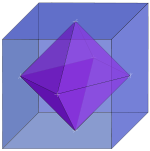A dual pair: cube and octahedron.

Every polyhedron has a dual (or "polar") polyhedron with faces and vertices interchanged. The dual of every Platonic solid is another Platonic solid, so that we can arrange the five solids into dual pairs.

• The tetrahedron is self-dual (i.e. its dual is another tetrahedron).
• The cube and the octahedron form a dual pair.
• The dodecahedron and the icosahedron form a dual pair.

If a polyhedron has Schläfli symbol {p, q}, then its dual has the symbol {q, p}. Indeed every combinatorial property of one Platonic solid can be interpreted as another combinatorial property of the dual.

One can construct the dual polyhedron by taking the vertices of the dual to be the centers of the faces of the original figure. The edges of the dual are formed by connecting the centers of adjacent faces in the original. In this way, the number of faces and vertices is interchanged, while the number of edges stays the same.

More generally, one can dualize a Platonic solid with respect to a sphere of radius d concentric with the solid. The radii (R, ρ, r) of a solid and those of its dual (R*, ρ*, r*) are related by$d^2 = R^\ast r = r^\ast R = \rho^\ast\rho.$

It is often convenient to dualize with respect to the midsphere (d = ρ) since it has the same relationship to both polyhedra. Taking d2 = Rr gives a dual solid with the same circumradius and inradius (i.e. R* = R and r* = r).

### Symmetry groups

In mathematics, the concept of symmetry is studied with the notion of a mathematical group. Every polyhedron has an associated symmetry group, which is the set of all transformations (Euclidean isometries) which leave the polyhedron invariant. The order of the symmetry group is the number of symmetries of the polyhedron. One often distinguishes between the full symmetry group, which includes reflections, and the proper symmetry group, which includes only rotations.

The symmetry groups of the Platonic solids are known as polyhedral groups (which are a special class of the point groups in three dimensions). The high degree of symmetry of the Platonic solids can be interpreted in a number of ways. Most importantly, the vertices of each solid are all equivalent under the action of the symmetry group, as are the edges and faces. One says the action of the symmetry group is transitive on the vertices, edges, and faces. In fact, this is another way of defining regularity of a polyhedron: a polyhedron is regular if and only if it is vertex-uniform, edge-uniform, and face-uniform.

There are only three symmetry groups associated with the Platonic solids rather than five, since the symmetry group of any polyhedron coincides with that of its dual. This is easily seen by examining the construction of the dual polyhedron. Any symmetry of the original must be a symmetry of the dual and vice-versa. The three polyhedral groups are:

• the tetrahedral group T,
• the octahedral group O (which is also the symmetry group of the cube), and
• the icosahedral group I (which is also the symmetry group of the dodecahedron).

The orders of the proper (rotation) groups are 12, 24, and 60 respectively — precisely twice the number of edges in the respective polyhedra. The orders of the full symmetry groups are twice as much again (24, 48, and 120). See (Coxeter 1973) for a derivation of these facts. All Platonic solids except the tetrahedron are centrally symmetric, meaning they are preserved under reflection through the origin.

The following table lists the various symmetry properties of the Platonic solids. The symmetry groups listed are the full groups with the rotation subgroups given in parenthesis (likewise for the number of symmetries). Wythoff's kaleidoscope construction is a method for constructing polyhedra directly from their symmetry groups. We list for reference Wythoff's symbol for each of the Platonic solids.

Polyhedron Schläfli symbol Wythoff symbol Dual polyhedron Symmetries Symmetry group
tetrahedron {3, 3} 3 | 2 3 tetrahedron 24 (12) Td (T)
cube {4, 3} 3 | 2 4 octahedron 48 (24) Oh (O)
octahedron {3, 4} 4 | 2 3 cube
dodecahedron {5, 3} 3 | 2 5 icosahedron 120 (60) Ih (I)
icosahedron {3, 5} 5 | 2 3 dodecahedron

## In nature and technology

The tetrahedron, cube, and octahedron all occur naturally in crystal structures. These by no means exhaust the numbers of possible forms of crystals. However, neither the regular icosahedron nor the regular dodecahedron are amongst them. One of the forms, called the pyritohedron (named for the group of minerals of which it is typical) has twelve pentagonal faces, arranged in the same pattern as the faces of the regular dodecahedron. The faces of the pyritohedron are, however, not regular, so the pyritohedron is also not regular.Circogonia icosahedra, a species of Radiolaria, shaped like a regular icosahedron.

In the early 20th century, Ernst Haeckel described (Haeckel, 1904) a number of species of Radiolaria, some of whose skeletons are shaped like various regular polyhedra. Examples include Circoporus octahedrus, Circogonia icosahedra, Lithocubus geometricus and Circorrhegma dodecahedra. The shapes of these creatures should be obvious from their names.

Many viruses, such as the herpes virus, have the shape of a regular icosahedron. Viral structures are built of repeated identical protein subunits and the icosahedron is the easiest shape to assemble using these subunits. A regular polyhedron is used because it can be built from a single basic unit protein used over and over again; this saves space in the viral genome.

In meteorology and climatology, global numerical models of atmospheric flow are of increasing interest which employ grids that are based on an icosahedron (refined by triangulation) instead of the more commonly used longitude/latitude grid. This has the advantage of evenly distributed spatial resolution without singularities (i.e. the poles) at the expense of somewhat greater numerical difficulty.

Geometry of space frames is often based on platonic solids. In MERO system, Platonic solids are used for naming convention of various space frame configurations. For example ½O+T refers to a configuration made of one half of octahedron and a tetrahedron.

Several Platonic hydrocarbons have been synthesised, including cubane and dodecahedrane.

Platonic solids are often used to make dice, because dice of these shapes can be made fair. 6-sided dice are very common, but the other numbers are commonly used in role-playing games. Such dice are commonly referred to as dn where n is the number of faces (d8, d20, etc.); see dice notation for more details.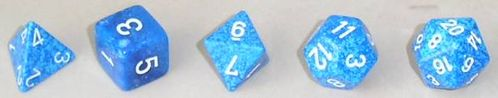Polyhedral dice are often used in role-playing games.

These shapes frequently show up in other games or puzzles. Puzzles similar to a Rubik's Cube come in all five shapes — see magic polyhedra.

### Liquid Crystals with symmetries of Platonic Solids

For the intermediate material phase called Liquid Crystals the existence of such symmetries was first proposed in 1981 by H. Kleinert and K. Maki and their structure was analyzed in. See the review article here. In aluminum the icosahedral structure was discovered three years after this by Dan Shechtman, which earned him the Nobel Prize in 2011.

## Related polyhedra and polytopes

### Uniform polyhedra

There exist four regular polyhedra which are not convex, called Kepler–Poinsot polyhedra. These all have icosahedral symmetry and may be obtained as stellations of the dodecahedron and the icosahedron.

The next most regular convex polyhedra after the Platonic solids are the cuboctahedron, which is a rectification of the cube and the octahedron, and the icosidodecahedron, which is a rectification of the dodecahedron and the icosahedron (the rectification of the self-dual tetrahedron is a regular octahedron). These are both quasi-regular, meaning that they are vertex- and edge-uniform and have regular faces, but the faces are not all congruent (coming in two different classes). They form two of the thirteen Archimedean solids, which are the convex uniform polyhedra with polyhedral symmetry.

The uniform polyhedra form a much broader class of polyhedra. These figures are vertex-uniform and have one or more types of regular or star polygons for faces. These include all the polyhedra mentioned above together with an infinite set of prisms, an infinite set of antiprisms, and 53 other non-convex forms.

The Johnson solids are convex polyhedra which have regular faces but are not uniform.

### Tessellations

The three regular tessellations of the plane are closely related to the Platonic solids. Indeed, one can view the Platonic solids as the five regular tessellations of the sphere. This is done by projecting each solid onto a concentric sphere. The faces project onto regular spherical polygons which exactly cover the sphere. One can show that every regular tessellation of the sphere is characterized by a pair of integers {p, q} with 1/p + 1/q > 1/2. Likewise, a regular tessellation of the plane is characterized by the condition 1/p + 1/q = 1/2. There are three possibilities:

In a similar manner one can consider regular tessellations of the hyperbolic plane. These are characterized by the condition 1/p + 1/q < 1/2. There is an infinite family of such tessellations.

### Higher dimensions

In more than three dimensions, polyhedra generalize to polytopes, with higher-dimensional convex regular polytopes being the equivalents of the three-dimensional Platonic solids.

In the mid-19th century the Swiss mathematician Ludwig Schläfli discovered the four-dimensional analogues of the Platonic solids, called convex regular 4-polytopes. There are exactly six of these figures; five are analogous to the Platonic solids, while the sixth one, the 24-cell, has one lower-dimension analogue (Truncation of a simplex-faceted polyhedron that has simplices for ridges and is self-dual): the Hexagon.

In all dimensions higher than four, there are only three convex regular polytopes: the simplex, the hypercube, and the cross-polytope. In three dimensions, these coincide with the tetrahedron, the cube, and the octahedron.

Wikimedia Foundation. 2010.

### Look at other dictionaries:

• Platonic solid — Geom. one of the five regular polyhedrons: tetrahedron, octahedron, hexahedron, icosahedron, or dodecahedron. [1950 55] * * * also known as regular polyhedron Geometric solid all of whose faces are identical regular polygons and all of whose… …   Universalium

• Platonic solid — noun any one of five solids whose faces are congruent regular polygons and whose polyhedral angles are all congruent • Syn: ↑regular polyhedron, ↑regular convex solid, ↑regular convex polyhedron, ↑Platonic body, ↑ideal solid • Hypernyms:… …   Useful english dictionary

• Platonic solid — noun Any one of the following five polyhedra: the regular tetrahedron, the cube, the regular octahedron, the regular dodecahedron and the regular icosahedro …   Wiktionary

• Platonic solid — (also Platonic body) noun one of five regular solids (a tetrahedron, cube, octahedron, dodecahedron, or icosahedron) …   English new terms dictionary

• platonic solid — /plətɒnɪk ˈsɒləd/ (say pluhtonik soluhd) noun any one of the five regular polyhedrons (tetrahedron, hexahedron, octahedron, dodecahedron, icosahedron) …   Australian English dictionary

• Platonic hydrocarbon — Platonic hydrocarbons are the molecular representation of platonic solid geometries with vertices replaced by carbon atoms and with edges replaced by chemical bonds. Not all platonic solids have a molecular counterpart:* Tetravalent carbon… …   Wikipedia

• Platonic body — noun any one of five solids whose faces are congruent regular polygons and whose polyhedral angles are all congruent • Syn: ↑regular polyhedron, ↑regular convex solid, ↑regular convex polyhedron, ↑Platonic solid, ↑ideal solid • Hypernyms:… …   Useful english dictionary

• Platonic — Plato s influence on Western culture was so profound that several different concepts are linked by being called platonic or Platonist, for accepting some assumptions of Platonism, but which do not imply acceptance of that philosophy as a whole.… …   Wikipedia

• solid — solidly, adv. solidness, n. /sol id/, adj. 1. having three dimensions (length, breadth, and thickness), as a geometrical body or figure. 2. of or pertaining to bodies or figures of three dimensions. 3. having the interior completely filled up,… …   Universalium

• Platonic — adjective Of or relating to the ancient Greek philosopher Plato or his philosophies. See Also: Plato, Platonically, Platonism, Platonist, Platonistic, Platonic body, Platonic hydrocarbon, Platonic solid …   Wiktionary